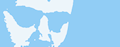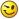## Physics Problem

18 replies [Last post]
Joined: 10/23/2009

Hi there I'm Texas a newbie at level creation.

I've got a major problem and I need guidance please.

It's the following: I made my level and when I'm trying to test it the level crashes imideatly after showing the screen with the name and subtitle of the level.

The crash reason that my computer says is something like: dMassCheck(mass)...ode\src\ode.cpp:472

Can anyone help?

Joined: 03/31/2009

It could be number of things! Something is wrong with your level, you made a mistake somewere. Go throw your game code or easier just start form scratch.

My Gooish profile | Videos on YouTube | My WOG Mods

Joined: 10/23/2009

Pavke wrote:
Go throw your game code or easier just start form scratch.

Well, I've built it from scratch anyway so I don't suppose there's a problem there.
About the code... where do I get it? I mean there are three bin files: the scenee one, the resrc one and the level...Which one should I post (or all)?

(thanks by the way for so promptly answering)Joined: 11/04/2008

If I remember correctly, dMassCheck errors are given when you have geometry with static=false but that doesn't have a mass.Joined: 04/10/2009

genius,pure genius

Crazeh man!

Joined: 10/23/2009

davidc wrote:
If I remember correctly, dMassCheck errors are given when you have geometry with static=false but that doesn't have a mass.

Thanks man. It was exactly that. I just needed to make them true instead of false.Joined: 07/21/2009

I haven't got any geom with "static - false" but I still have this problem.

Joined: 10/23/2009

For some reason my problem came back...even after I've turned all static=false into static=true...

the oddest thing is that for some time I actually entered the level.(It had a lot of problems in it though but I still managed to enter)

How come is this possible?

Edit:

OK my bad! didn't read all of your instructions. I should't change the option to false but instead to give mass a number.

Now it works at least. thanks davidcJoined: 11/04/2008

static=false (or if static is not set at all) means that it's dynamic geometry. It can move around within the level, it therefore needs a mass to determine how it reacts.

static=true is, so to speak, "fixed to the wall". It can't move at all, so it doesn't need a mass.

Martin33, you may not have static=false but do you have any geometries (or composite geometries) that you haven't set static on at all?Joined: 11/04/2008

You should also avoid positioning geometries at the exact same position as forcefields, this seems to cause weird effects. Move them apart by a small amount instead.

Joined: 10/23/2009

I'm making a wheel that sould spin nailed to the backgound. If I turn static to false the wheel falls, if I turn it to true it doesn't spin any more.

What should I do?Joined: 11/04/2008

Check out the scene of http://goofans.com/download/sample/rotating-planet for the simplest example of this.

Joined: 10/23/2009

will do thxJoined: 07/21/2009

davidc wrote:
static=false (or if static is not set at all) means that it's dynamic geometry. It can move around within the level, it therefore needs a mass to determine how it reacts.

static=true is, so to speak, "fixed to the wall". It can't move at all, so it doesn't need a mass.

Martin33, you may not have static=false but do you have any geometries (or composite geometries) that you haven't set static on at all?

only in compozitegeoms.
whole group of geometries has got static=true, but single geoms inside composite.Joined: 07/21/2009

Can someone help me with this?Joined: 11/04/2008

Maybe post your decrypted scene file here so we can see it?Joined: 07/21/2009

```- <scene backgroundcolor="0,0,0" maxx="1300" maxy="1600" minx="-500" miny="60">
<linearforcefield antigrav="true" dampeningfactor="0" force="20,-10" geomonly="false" type="gravity" />
<line anchor="1330,300" id="right" material="rock" normal="-1,0" static="true" tag="detaching" />
<line anchor="-500,300" id="left" material="rock" normal="1,0" static="true" tag="detaching" />
<line anchor="0,20" id="ground" material="rock" normal="0,1" static="true" tag="deadly" />
<SceneLayer depth="0" id="main" image="LEVEL_IMAGE_MAIN" scalex="1.5" scaley="1.5" x="-171.98287319" y="354.538502246" />
<SceneLayer depth="0" id="leb" image="LEVEL_IMAGE_GLOWA" rotation="0" scalex="1.5" scaley="1.5" x="904.890705817" y="1158.3925288" />
<SceneLayer depth="-30" id="jezyk" image="LEVEL_IMAGE_JEZYK" rotation="42.8549344964" scalex="1.5" scaley="1.5" x="1060.70326303" y="1031.82028308" />
<SceneLayer depth="-1" id="reka" image="LEVEL_IMAGE_REKA" rotation="13.1044027958" scalex="2" scaley="2" x="954.30632678" y="253.211257912" />
<SceneLayer depth="-1" image="bush1" rotation="23.7157404163" scalex="2" scaley="2" x="-250.230881178" y="730.19689216" />
<SceneLayer depth="-2" image="bush2" rotation="15.9447326362" scalex="1.5" scaley="2" x="-133.0" y="791.0" />
<SceneLayer colorize="128,128,128" depth="-1.5" image="bush1" rotation="5.32466019507" scalex="1.5" scaley="1.5" x="-269.0" y="788.0" />
<SceneLayer depth="-5" image="tree_stripe1" rotation="29.8617462718" scalex="2" scaley="2" x="-333.44" y="1166.64" />
<SceneLayer depth="-2" id="kolce" image="spikeGray_group" rotation="-128.113771824" scalex="1" scaley="1.3" x="165.824" y="441.6672" />
<SceneLayer depth="-3" id="kolce" image="spikeGray_group" rotation="-90.7467768695" scalex="1" scaley="1.2" x="71.0861106382" y="197.169875676" />
<SceneLayer depth="-2" id="kolce" image="spikeGray_group" rotation="-63.2528762137" scalex="1" scaley="1.3" x="163.629021884" y="131.648307161" />
- <compositegeom id="head" material="rock" rotation="0.0" static="true" x="0" y="0">
<circle id="geometry1" radius="67.1933297225" x="616.226088284" y="1093.96729847" />
<rectangle height="140" id="geometry2" rotation="0.941237420381" width="300" x="721.93178525" y="1257.13134393" />
<rectangle height="70" id="geometry3" rotation="-0.390763907809" width="30" x="580.383449326" y="1140.32883762" />
<rectangle height="100" id="geometry4" rotation="0.665092107667" width="300" x="912.128633622" y="1414.79135327" />
<rectangle height="100" id="geometry5" rotation="0.0" width="200" x="1101.144641" y="1480.38501561" />
<rectangle height="200" id="geometry6" rotation="0.364640462215" width="100" x="1234.23878958" y="1373.68362695" />
<rectangle height="200" id="geometry7" rotation="0.0" width="100" x="1254.07431126" y="1221.42137873" />
<rectangle height="140" id="geometry8" rotation="0.629623793895" width="500" x="1182.86809246" y="999.908312366" />
<rectangle height="150" id="geometry9" rotation="-0.496743054025" width="80" x="1205.37286652" y="1092.04576714" />
<circle id="geometry10" radius="72.3447922802" x="978.578477487" y="852.941232324" />
</compositegeom>
- <compositegeom id="hand" material="rock" rotation="0.0" static="true" x="0" y="0">
<rectangle height="130" id="geometry11" rotation="-0.252085955008" width="500" x="1066.9937565" y="54.1331945888" />
<circle id="geometry12" radius="46.5398267871" x="823.716055185" y="90.8959752945" />
<rectangle height="500" id="geometry13" rotation="0.452566906731" width="110" x="788.102111376" y="298.697593234" />
<rectangle height="400" id="geometry14" rotation="0.328932302354" width="100" x="763.976536537" y="282.50150345" />
<rectangle height="70" id="geometry15" rotation="0.477015370646" width="30" x="624.371932998" y="527.759651344" />
<rectangle height="30" id="geometry16" rotation="0.173542438224" width="80" x="585.028154521" y="549.164359607" />
<rectangle height="120" id="geometry17" rotation="0.371935083888" width="30" x="664.287141277" y="569.008992792" />
<rectangle height="30" id="geometry18" rotation="-0.524143873383" width="50" x="626.064979707" y="628.319872527" />
<rectangle height="150" id="geometry19" rotation="0.228848391516" width="25" x="713.279633103" y="597.283994409" />
<rectangle height="150" id="geometry20" rotation="-0.64368940781" width="30" x="771.277452451" y="553.785629897" />
<rectangle height="100" id="geometry21" rotation="0.72354729505" width="25" x="782.97056119" y="635.169666724" />
</compositegeom>
<rectangle height="100" id="geometry22" rotation="0.961207244883" width="200" x="670.990206379" y="1128.20407586" />
<rectangle height="100" id="geometry23" rotation="0.66079967626" width="200" x="990.624591706" y="895.109126319" />
<rectangle height="100" id="geometry24" rotation="1.0815562028" width="300" x="755.572330311" y="1365.84158256" />
<circle id="geometry25" radius="129.024693607" x="845.623430865" y="1552.0296285" />
<circle id="geometry31" radius="149.509685873" x="61.9217060999" y="534.67011613" />
<rectangle height="200" id="geometry32" rotation="0.655303350653" width="100" x="19.798020643" y="226.539157303" />
<rectangle height="130" id="geometry33" rotation="-0.651452597144" width="100" x="13.5321441851" y="379.584079638" />
<circle id="geometry26" radius="298.056334857" x="-96.0617183696" y="-25.2712932455" />
<rectangle height="100" id="geometry27" rotation="0.509034571018" width="500" x="1103.75462909" y="1756.5466526" />
<rectangle height="10" id="geometry28" rotation="0.468570965927" width="40" x="529.351005465" y="536.102978626" />
<rectangle height="10" id="geometry29" rotation="0.0" width="40" x="584.111689247" y="641.124432396" />
<rectangle height="40" id="geometry30" rotation="0.840812686821" width="10" x="679.850069252" y="680.38792532" />
<rectangle height="10" id="geometry40" rotation="-0.400313855447" width="40" x="735.787100266" y="677.698644983" />
</compositegeom>
<circle id="geometry34" material="rock" radius="144.635392562" x="83.6396671213" y="602.489127572" />
<rectangle height="100" id="geometry35" material="rock" rotation="-0.324579807795" width="100" x="63.1933499921" y="708.81073832" />
<rectangle height="100" id="geometry36" material="rock" rotation="0.0316693863569" width="100" x="-11.8888474132" y="719.159440646" />
<rectangle height="100" id="geometry37" material="rock" rotation="0.325592577424" width="100" x="-91.1402002159" y="703.713979727" />
<rectangle height="100" id="geometry38" material="rock" rotation="0.11213548718" width="200" x="-212.983765267" y="678.885751792" />
<rectangle height="100" id="geometry39" material="rock" rotation="-0.0541138965774" width="250" x="-408.871797299" y="673.994455495" />
<SceneLayer depth="-100" id="bg" image="tlo" scalex="8" scaley="8" x="422.541560688" y="829.957736631" />
<particles depth="-0.8" effect="gooDrips" pos="841.053775131,803.759374798" pretick="850" />
<particles depth="400" effect="blackBallsRising" pos="302.12031191,1901.39451421" pretick="0" />
</scene>```

here is scene.bin content

or scene, level and resources files here

level is not finished yet, but I can't continue doing it when i can't test if it works good.Joined: 11/04/2008

geometry34-39 all need either static="true" or a mass.Joined: 07/21/2009

Thank you!
I haven't noticed this
now level works